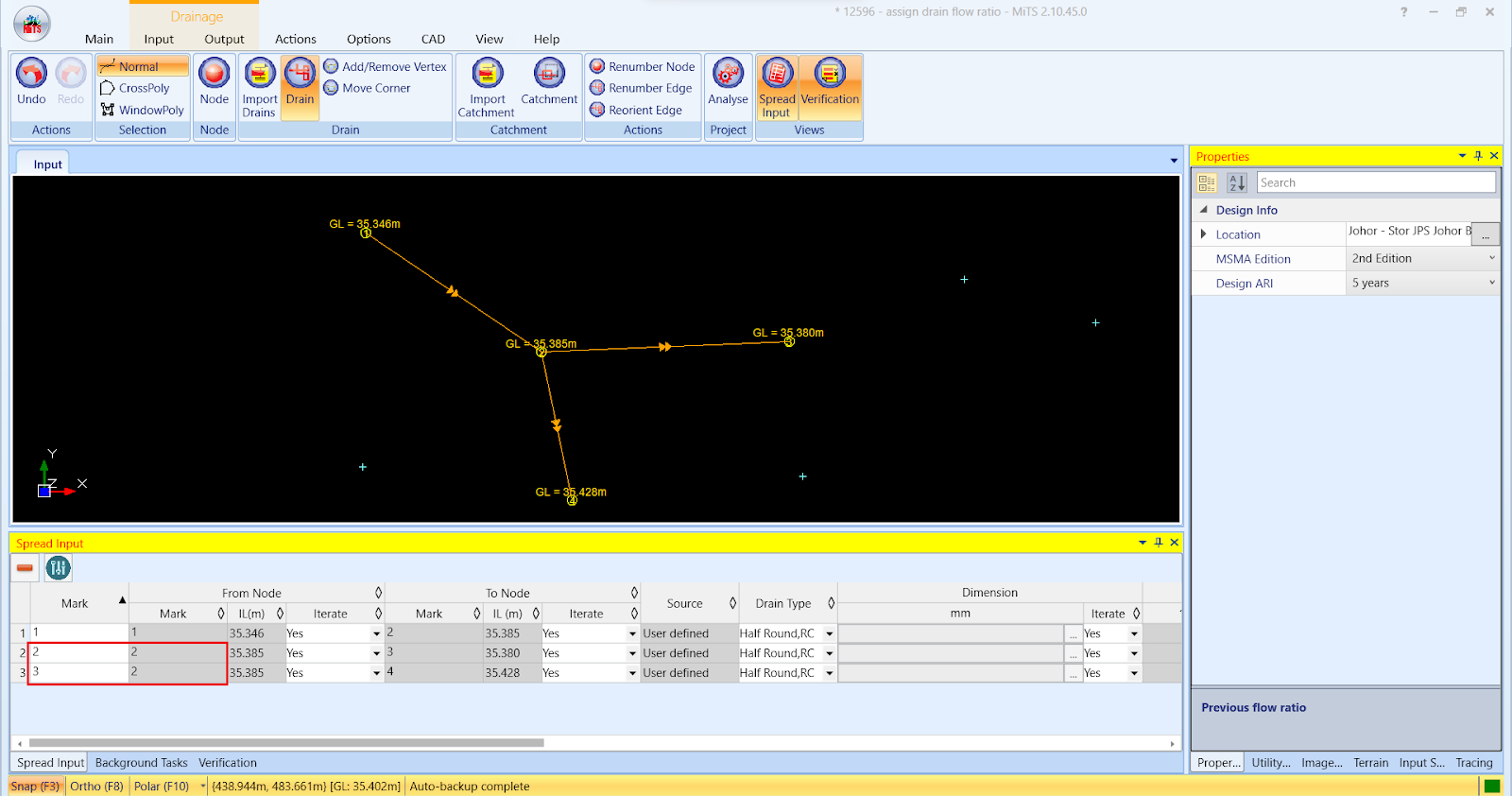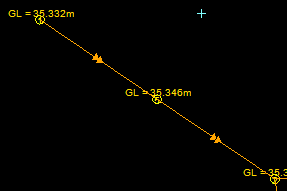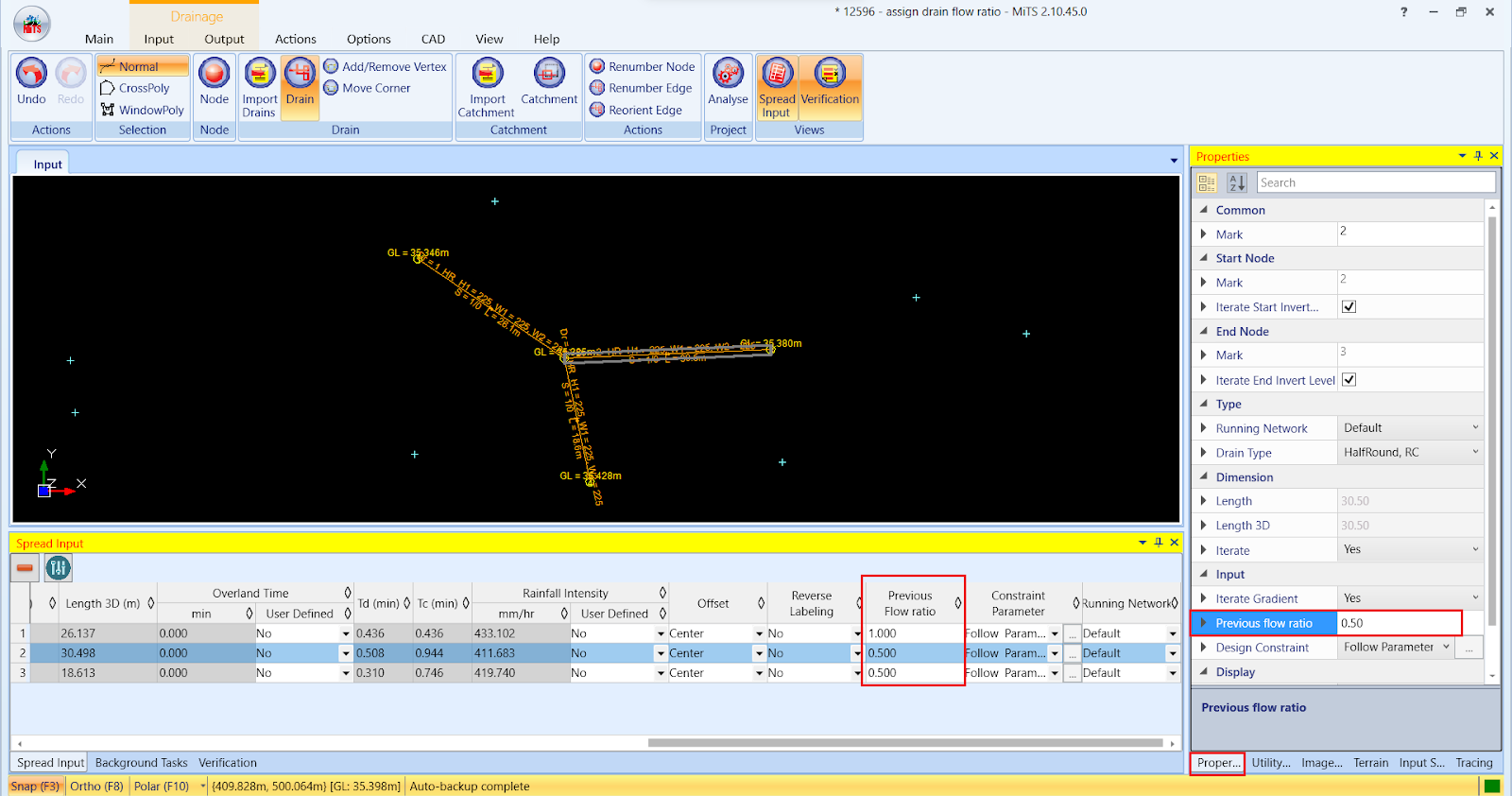603 - 5885 1250 (4 lines) info@mes100.com
Select Page

# What is Flow Ratio?

In designing drainage, the flow ratio is one of the most important factors as it determines how the flow is distributed between two or more outgoing drains. When a drain is connected to two or more drains, the ratio of the flows that go into each drain is called Flow Ratio. Obviously, the summation of the flow ratio of these two drains must be 1 (the conservation of charge), because the charge amount that comes from the incoming drains must be the same.

As shown in the image below, the flow ratio is divided into two different directions. In this case, each drain (drain 2&3) receives a 0.5 flow ratio. Users can change the flow ratio so that the distribution of flow is uneven for the two outgoing drains (eg: 0.6 and 0.4).The actual flow distribution can be auto-calculated from Kinematic Wave or Full dynamic wave routing, this is something that we aim to improve in the future. For the time being, we just let users set the flow ratio distribution.

When you have only one outgoing drain, the flow ratio must be equivalent to 1. As shown in the image below, both drainage shows only one outgoing drain. Thus, the flow ratio is equal to 1.In the MiTS 2 software, the flow ratio is shown in spread input table and RHS panel in the “Properties” tab under the “Previous Flow Ratio” column/row as per the image below. Users can adjust the flow ratio here.